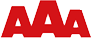Sales tax calculator

When you buy a product, some of the money usually goes to sales tax. We built this spreadsheet to let you enter the amount before tax, and the sales tax rate. The tax and total are calculated automatically.

Test this calculator in other formats:

Home Equity calculator with live interactive chart

This is one of our favorite examples because it shows the advantage of live charts. Change any cell in the spreadsheet and the chart immediately reflects the new values.

In this example, you can also see a useful implementation of a slider. To change the years remaining on a loan, we could just have used a standard numeric field. Using a slider allows for much easier visualization of how the equity varies over the lifetime of the loan.

Equated Monthly Installment calculator

An Equal Monthly Installment is a fixed amount returned from the borrower to the lender each month. This means that both the amount returned from the principal loan and the interest is fixed so that over a specified time the whole loan is paid back in full.

We created an EMI spreadsheet that shows borrowers the monthly payment and the time period allowed paying off the loan, so they can take the right financial move by planning a proper budget. The spreadsheet also shows the total cost of the loan.

Test this calculator in other formats:

Bond Price calculator

A bond is a long-term promissory note issued by a business or a business firm. The value of the bond price is the sum of present value of annuities (or coupon) over the life of the bond and present value of the face value of the bond. The bond value or price of the bond is calculated on the basis of face value, coupon rate, market rate and maturity period of the bond.

As an example, a company issues seven-year bond with coupon rate of 14% payable semiannually. The face value of the bond is \$1,000.00. The market rate of interest is 14%. To calculate the price of the bond, we created this spreadsheet.

Test this calculator in other formats:

Beta Risk calculator

We created a spreadsheet that tries to find out the effect of leverage on the systematic risk of a company’s stock returns. It is trying to explore the effect of employing various levels of Debt in the Capital Structure of the firm, on the risk of the company’s returns.

Test this calculator in other formats: